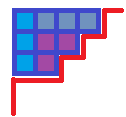## LOJ3153 「JOI Open 2019」三级跳

• 是合法的当且仅当 L \le A < B < C \le R(B-A) \leq (C-B)
• 的权值为 a_A + a_B + a_C

## LOJ6051 「雅礼集训 2017 Day11」PATH

n, a_i \leq 5\times 10^5

## BZOJ4401 块的计数

#### 暴力 DP

DFS 自底向上计算，用 dp_u 表示到 u 为止，未被分块的节点个数。

## NOI 模拟赛 20190711A 刀剑传奇

#### Solution1 - 二元生成函数

\forall \; i , \; [y^k \cdot x^i] \prod_{\textrm{or}} 1 + y x^{a_i}

[x^k] \operatorname{FWT_{\textrm{or}}} (F_i(x)) = \begin{cases} 1 & (a_i \notin k) \\ 1 + y & (a_i \in k) \\ \end{cases}

\theta 也是好求的，每一个 F_i(x) = 1 + y x^{a_i} 只会对答案贡献 1 ，且一定是在 a_i \in k 的位置。可以直接定义 G(x) = \sum_{i=0}^\infty x^i \sum_{j=1}^n [a_j = i] ，然后做一次高维前缀和。

#### Solution2 - 容斥

cnt_S = \sum_{i=1}^n [a_i \cup S = \emptyset] ，即与 S 没有交集的 a_i 个数。

\operatorname{query}(S) = \sum_{T \in S} (-1)^{|T|} \binom {cnt_T} k

## NOI 模拟赛 20190711B 三格骨牌before transformation after transformation image
0001 10000011 10100101 11000111 1110## LOJ575 「LibreOJ NOI Round #2」不等关系

f_{i, j} = \begin{cases} \displaystyle \sum_{k=1}^{i-1} f_{i-1, k} & (s_{i - 1} = \textrm{<}) \\ \displaystyle \sum_{k=i}^j f_{i-1, k} & (s_{i - 1} = \textrm{>}) \\ \end{cases}

dp_n = \begin{cases} 1 & (n = 0) \\ \displaystyle \sum_{j=0}^{i-1} [j = 0 \textrm{ or } s_j = \textrm{<}] (-1)^{cnt_{i-1} - cnt_j} \frac {dp_{j}} {(i - j)!} & (n > 0) \\ \end{cases}

## LOJ573 「LibreOJ NOI Round #2」单枪匹马

\left[\begin{matrix} a & 1 \\ 1 & 0 \\ \end{matrix}\right] \left[\begin{matrix} x \\ y \\ \end{matrix}\right] = \left[\begin{matrix} ax + y \\ x \end{matrix}\right]

\left[\begin{matrix} 0 & 1 \\ 1 & -a \\ \end{matrix}\right] \left[\begin{matrix} ax + y \\ x \\ \end{matrix}\right] = \left[\begin{matrix} x \\ y \\ \end{matrix}\right]

\begin{aligned} Ans_{l, r} &= \left[\begin{matrix} a_l & 1 \\ 1 & 0 \\ \end{matrix}\right] \left[\begin{matrix} a_{l + 1} & 1 \\ 1 & 0 \\ \end{matrix}\right] \cdots \left[\begin{matrix} a_r & 1 \\ 1 & 0 \\ \end{matrix}\right] \left[\begin{matrix} 1 \\ 0 \end{matrix}\right] \\ &= \left[\begin{matrix} 0 & 1 \\ 1 & -a_{l - 1} \\ \end{matrix}\right] \left[\begin{matrix} 0 & 1 \\ 1 & -a_{l - 2} \\ \end{matrix}\right] \cdots \left[\begin{matrix} 0 & 1 \\ 1 & -a_1 \\ \end{matrix}\right] \left[\begin{matrix} a_1 & 1 \\ 1 & 0 \\ \end{matrix}\right] \left[\begin{matrix} a_2 & 1 \\ 1 & 0 \\ \end{matrix}\right] \cdots \left[\begin{matrix} a_r & 1 \\ 1 & 0 \\ \end{matrix}\right] \left[\begin{matrix} 1 \\ 0 \end{matrix}\right] \end{aligned}

\begin{aligned} F_n &= \left[\begin{matrix} a_1 & 1 \\ 1 & 0 \\ \end{matrix}\right] \left[\begin{matrix} a_2 & 1 \\ 1 & 0 \\ \end{matrix}\right] \cdots \left[\begin{matrix} a_n & 1 \\ 1 & 0 \\ \end{matrix}\right] \\ G_n &= \left[\begin{matrix} 0 & 1 \\ 1 & -a_{n} \\ \end{matrix}\right] \left[\begin{matrix} 0 & 1 \\ 1 & -a_{n - 1} \\ \end{matrix}\right] \cdots \left[\begin{matrix} 0 & 1 \\ 1 & -a_1 \\ \end{matrix}\right] \end{aligned}

## 洛谷4643 [国家集训队]阿狸和桃子的游戏

• 如果两边都是黑点，对答案的贡献为 1 ，可以看做两个黑点分别为答案贡献了 \frac 1 2
• 如果两边都是白点，对答案的贡献为 -1 ，可以看做两个白点分别为答案贡献了 - \frac 1 2
• 如果一边是白点一边是黑点，贡献为 0 ，可以看做黑点为答案贡献了 \frac 1 2 的边权，白点为答案贡献了 - \frac 1 2 的贡献

## LOJ6674 赛道修建

\clubsuit 是 OI 国马拉松协会的会长。有一天，他打算举办全国青少年马拉松奥林匹克联赛（National Olympiad in Marathon in Provinces, NOMP），于是准备在 OI 国中修建一条赛道。

OI 国有 n (n \leq 5 \times 10^4) 个城市，一共有 n - 1 条道路将它们连通。也就是说，OI 国的结构是一棵树。

\clubsuit 会选择一个城市作为起点，再选择一个城市作为终点，这样赛道的长度就等于起点到终点路径上的边数。由于 OI 国的群众非常懒，所以赛道长为 0 也是允许的。